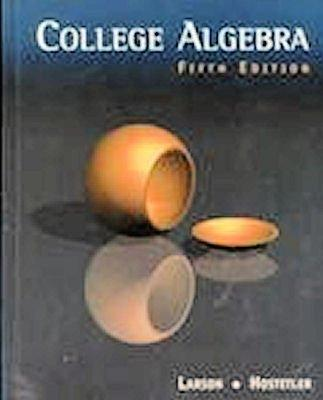114163

9780618052844

# College Algebra, Fifth Edition\$3.09
\$3.95 Shipping
List Price
\$216.95
Discount
98% Off
You Save
\$213.86

• Condition: Good
• Provider: Gulf Coast Books Contact
• Provider Rating:
87%
• Ships From: Memphis, TN
• Shipping: Standard30-day money back guarantee

Most renters respond to questions in 48 hours or less.
The response will be emailed to you.
• ISBN-13: 9780618052844
• ISBN: 0618052844
• Edition: 5
• Publication Date: 2000
• Publisher: Brooks Cole

Ron Larson

### SUMMARY

Contents Note: Each chapter contains a Summary, Review Exercises, and a Test. An Introduction to Graphing Utilities P. Prerequisites P.1 Real Numbers P.2 Exponents and Radicals P.3 Polynomials and Special Products P.4 Factoring P.5 Rational Expressions P.6 Errors and the Algebra of Calculus P.7 Graphical Representation of Data Project: Numerically Finding a Maximum Volume 1. Equations and Inequalities 1.1 Graphs of Equations 1.2 Linear Equations in One Variable 1.3 Modeling with Linear Equations 1.4 Quadratic Equations 1.5 Complex Numbers 1.6 Other Types of Equations 1.7 Linear Inequalities 1.8 Other Types of Inequalities Project: Finding Falling Times Numerically 2. Functions and Their Graphs 2.1 Linear Equations in Two Variables 2.2 Functions 2.3 Analyzing Graphs of Functions 2.4 Shifting, Reflecting, and Stretching Graphs 2.5 Combinations of Functions 2.6 Inverse Functions Project: A Graphical Approach to Maximization Cumulative Test for Chapters P-2 3. Polynomial Functions 3.1 Quadratic Functions 3.2 Polynomial Functions of Higher Degree 3.3 Polynomial and Synthetic Division 3.4 Zeros of Polynomial Functions 3.5 Mathematical Modeling Project: Finding Points of Intersection Graphically 4. Rational Functions and Conics 4.1 Rational Functions and Asymptotes 4.2 Graphs of Rational Functions 4.3 Partial Fractions 4.4 Conics 4.5 Translations of Conics Project: Graphical Approach to Finding Average Cost 5. Exponential and Logarithmic Functions 5.1 Exponential Functions and Their Graphs 5.2 Logarithmic Functions and Their Graphs 5.3 Properties of Logarithms 5.4 Exponential and Logarithmic Equations 5.5 Exponential and Logarithmic Models Project: Graphical Approach to Compound Interest Cumulative Test for Chapters 3-5 6. Systems of Equations and Inequalities 6.1 Solving Systems of Equations 6.2 Two-Variable linear Systems 6.3 Multivariable Linear Systems 6.4 Systems of Inequalities 6.5 Linear Programming Project: Fitting Models to Data 7. Matrices and Determinants 7.1 Matrices and Systems of Equations 7.2 Operations with Matrices 7.3 The Inverse of a Square Matrix 7.4 The Determinant of a Square Matrix 7.5 Applications of Matrices and Determinants Project: Solving Systems of Equations 8. Sequences, Series, and Probability 8.1 Sequences and Series 8.2 Arithmetic Sequences and Partial Sums 8.3 Geometric Sequences and Series 8.4 Mathematical Induction 8.5 The Binomial Theorem 8.6 Counting Principles 8.7 Probability Project: Exploring Difference Quotients Cumulative Test for Chapters 6-8 Appendices A. Proofs of Selected Theorems B. Concepts in Statistics. B.1 Representing Data. B.2 Measures of Central Tendency and Dispersion. B.3 Least Squares RegressionRon Larson is the author of 'College Algebra, Fifth Edition', published 2000 under ISBN 9780618052844 and ISBN 0618052844.

You can find lots of answers to common customer questions in our FAQs

View a detailed breakdown of our shipping prices